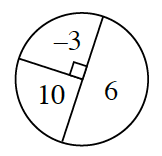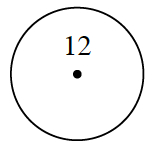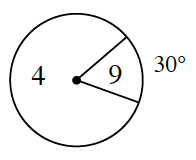### Home > GC > Chapter 10 > Lesson 10.2.3 > Problem10-81

10-81.

For each spinner below, find the expected value of one spin.

1.1.1.$\frac{1}{4}\left(-3\right)+\frac{1}{4}\left(10\right)+\frac{1}{2}\left(6\right)=\frac{19}{4}$

$12$

What is the measure of the unmarked arc?